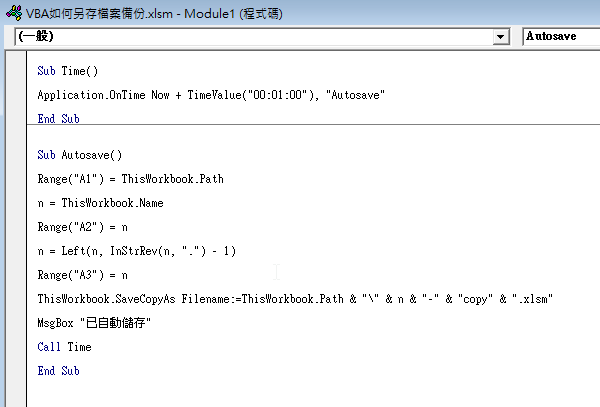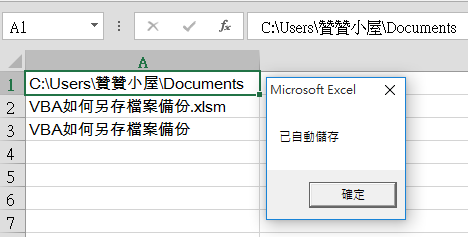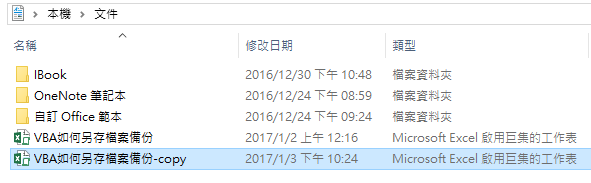• 文章分類

# VBA如何另存檔案備份Sub Autosave()

Range(“A1”) = ThisWorkbook.Path

n = ThisWorkbook.Name

Range(“A2”) = n

n = Left(n, InStrRev(n, “.”) – 1)

Range(“A3”) = n

ThisWorkbook.SaveCopyAs Filename:=ThisWorkbook.Path & “\” & n & “-” & “copy” & “.xlsm”

MsgBox “已自動儲存”

Call Time

End Sub